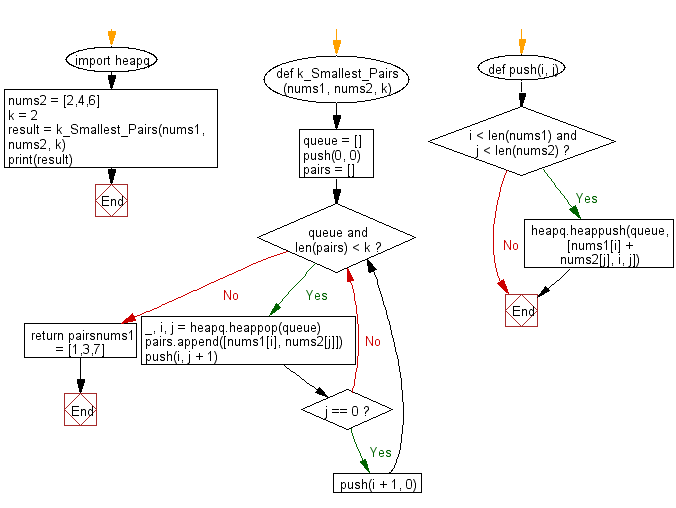﻿ Python heap queue algorithm: Find k number of pairs which consists of one element from the first array and one element from the second array - w3resource

# Python: Find k number of pairs which consists of one element from the first array and one element from the second array

## Python heap queue algorithm: Exercise-17 with Solution

You have two integer arrays sorted in ascending order and an integer k. Write a Python program to find k number of pairs (u, v) which consist of one element from the first array and one element from the second array using the heap queue algorithm.

Sample Solution:

Python Code:

``````import heapq
def k_Smallest_Pairs(nums1, nums2, k):
queue = []
def push(i, j):
if i < len(nums1) and j < len(nums2):
heapq.heappush(queue, [nums1[i] + nums2[j], i, j])
push(0, 0)
pairs = []
while queue and len(pairs) < k:
_, i, j = heapq.heappop(queue)
pairs.append([nums1[i], nums2[j]])
push(i, j + 1)
if j == 0:
push(i + 1, 0)
return pairs
nums1 = [1,3,7]
nums2 = [2,4,6]
k = 2
result = k_Smallest_Pairs(nums1, nums2, k)
print(result)
```
```

Sample Output:

```[[1, 2], [1, 4]]
```

Flowchart:Python Code Editor:

Have another way to solve this solution? Contribute your code (and comments) through Disqus.

What is the difficulty level of this exercise?

Test your Programming skills with w3resource's quiz.

﻿